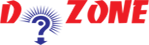﻿ Python Program to Solve Quadratic Equation## Python Program to Solve Quadratic Equation

This program evaluates roots of quadratic equation when coefficients a, b and c are known. The standard form of a quadratic equation is:

``````
ax2 + bx + c = 0, where
a, b and c are real numbers and
a ≠ 0
```
```
``````
Input
# Solve the quadratic equation ax**2 + bx + c = 0

# import complex math module
import cmath

a = 1
b = 5
c = 6

# To take coefficient input from the users
# a = float(input('Enter a: '))
# b = float(input('Enter b: '))
# c = float(input('Enter c: '))

# calculate the discriminant
d = (b**2) - (4*a*c)

# find two solutions
sol1 = (-b-cmath.sqrt(d))/(2*a)
sol2 = (-b+cmath.sqrt(d))/(2*a)

print('The solution are {0} and {1}'.format(sol1,sol2))
Output

Enter a: 1
Enter b: 5
Enter c: 6
The solutions are (-3+0j) and (-2+0j)
```
```

Conclusion: We have applied the cmath module to perform complex square root. Firstly, calculate the discriminant and then find the two solutions of the quadratic equation. You can also change the value of a, b and c in the above program to test it.

## Other Python Programs

PythonTraining, 258-FF , Katewa Nagar, New Sanganer Rd, Jaipur, 302019